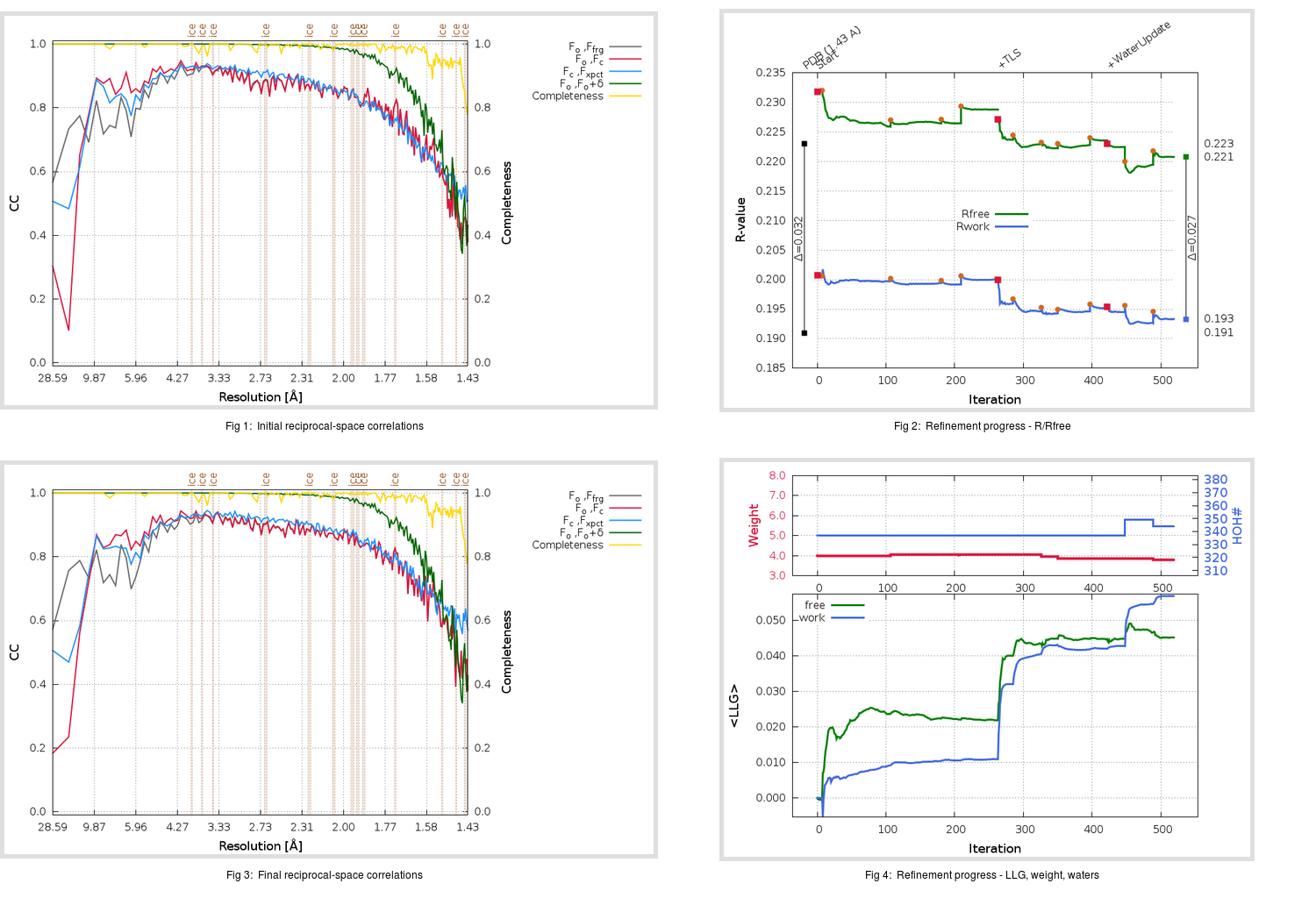Content:

## Deposited

` `
 Date deposited Date data collection Resolution R, Rfree 20200315 20200305 1.43 0.1900 0.2230

Molprobity (CCP4 7.0 version) summary:

```Ramachandran outliers =   0.33 %
favored =  98.34 %
Rotamer outliers      =   1.52 %
C-beta deviations     =     0
Clashscore            =   5.27
RMS(bonds)            =   0.0135
RMS(angles)           =   1.90
MolProbity score      =   1.42
Resolution            =   1.43
R-work                =   0.1900
R-free                =   0.2230
```

```Number of waters      =   337

<B> (all atoms) =   21.22 ( sd =    8.99 ) for       2738 non-hydrogen atoms
<B>   (protein) =   19.78 ( sd =    8.03 ) for       2370 non-hydrogen atoms
<B>     (water) =   30.61 ( sd =    9.20 ) for        337 non-hydrogen atoms
<B>    (others) =   27.09 ( sd =   10.60 ) for         31 non-hydrogen atoms

B min/max       (all non-hydrogen atoms) =    9.37 /   70.85
B min/max   (protein non-hydrogen atoms) =    9.37 /   66.13
B min/max     (water non-hydrogen atoms) =   12.38 /   70.85
B min/max     (other non-hydrogen atoms) =   14.08 /   44.11
```

## BUSTER (re-)refinement

` `

Molprobity (CCP4 7.0 version) summary:

```Ramachandran outliers =   0.33 %
favored =  98.68 %
Rotamer outliers      =   0.76 %
C-beta deviations     =     0
Clashscore            =   1.69
RMS(bonds)            =   0.0115
RMS(angles)           =   1.61
MolProbity score      =   0.92
Resolution            =   1.43
R-work                =   0.1933
R-free                =   0.2208
```

```Number of waters      =   344

<B> (all atoms) =   23.06 ( sd =    8.79 ) for       2745 non-hydrogen atoms
<B>   (protein) =   21.41 ( sd =    7.35 ) for       2370 non-hydrogen atoms
<B>     (water) =   33.76 ( sd =    9.72 ) for        344 non-hydrogen atoms
<B>    (others) =   28.22 ( sd =   10.76 ) for         31 non-hydrogen atoms

B min/max       (all non-hydrogen atoms) =    7.17 /   67.38
B min/max   (protein non-hydrogen atoms) =    7.17 /   59.79
B min/max     (water non-hydrogen atoms) =   11.80 /   67.38
B min/max     (other non-hydrogen atoms) =   16.44 /   41.69
```

Refinement progression:Results:

` `
 File Remark 5RFW_aB_refine.01_03_refine.pdb.gz exact refinement commands are in header 5RFW_aB_refine.01_03_refine.mtz.gz including original deposited data and several re-refinement map coefficients 5RFW_aB_refine.01_03_BUSTER_model.cif.gz including any non-standard compound restraints 5RFW_aB_refine.01_03_BUSTER_refln.cif.gz